# Gini Index

## Definition

For the purpose of measuring concentration, the Gini Index (also Gini coefficient) is an index defined in terms of the Lorentz curve of distribution values.

## Details

More precisely, if we have n values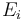$E_i$ summing up to a total value of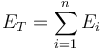$E_T = \sum^{n}_{i=1} E_{i}$

and the fractional value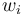$w_i$ is defined as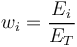$w_{i} = \frac{E_i}{E_T}$

Then the Gini index is defined as the area under the Lorenz curve which is geometrically reduced to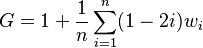$G = 1 + \frac{1}{n} \sum^{n}_{i=1} (1 - 2 i) w_{i}$

## Alternative Formula

Gini's absolute mean difference is defined as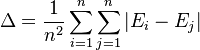$\Delta = \frac{1}{n^2} \sum^{n}_{i=1} \sum^{n}_{j=1} | E_i - E_j |$

The relative mean difference is defined as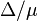$\Delta / \mu$ where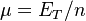$\mu = E_T / n$

The Gini index is equivalently given by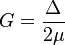$G = \frac{\Delta}{2 \mu}$

None

None

## Issues and Challenges

NB: Sometimes the formula appears also with the opposite sign!

## Implementations

Open Source implementations of the Gini index are available in